### Introduction

When an object's velocity chages, it accelerates. Acceleration shows the change in velocity in a unit time. Velocity is measured in meters per second, m/s, so acceleration is measured in (m/s)/s, or m/s2, which can be both positive and negative

The average acceleration is the ratio between the change in velocity and the time interval:#### Example

If a car moves from the rest to 5m/s in 5 seconds, its average acceleration is:An instantaneous acceleration is the change in velocity at one moment.

#### Velocity-time Graph

Let's examine more about a velocity-time graph. We can say that the tangent of a velocity-time graph represents instantaneous acceleration sinceFor example, the instantaneous acceleration when t = 3 at the below graph is 3 m/s2, since the graph has a slope of 3 when t = 3.#### Displacement and Constant Acceleration

In this section, we will assume that acceleration is always constant. We know that the area under the line of a velocity-time graph represents the displacement. Therefore, the equation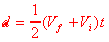is true, where Vi is the initial velocity and Vf is the final velocity, since the area of a triangle is 1/2 * width * height.The final velocity of a uniformly accelerated object iswhere:

• Vf is the final velocity in m/s,
• Vi is the initial velocity in m/s,
• a is acceleration in m/s2, and
• t is time in second.

Therefore, by substituting it to the previous equation,therefore,is true. If you don't understand the derivation, don't worry. The red formulae are the ones that you should learn.

From equations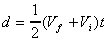and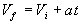, we can also say that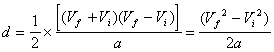Therefore,is true. These four red equations are very important and you should be very familiar with them. (It doesn't mean that you should memorize these formulae. Learn by using them.)

#### Acceleration Due to Gravity

Galileo was the first to find out that all objects falling to Earth have a constant acceleration of 9.80 m/s2 regardless of their mass. Acceleration due to gravity is given a symbol g, which equals to 9.80 m/s2.

Therefore, if you drop a pen, it should behave like this...

Time (s) Velocity (m/s) Displacement (m)
0 0 0
1 9.8 4.9
2 19.6 19.6
3 29.4 44.1
4 39.2 78.4

For all previous equations, we can substitute g for a: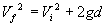### References & Resources

• http://library.thinkquest.org/10796/ch2/ch2.htm